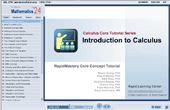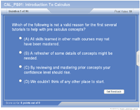HOME COURSES PREVIEW REVIEW ABOUT CONTACTTOLL-FREE INFO & ORDERING: M-F: 9am-5pm (PST): (877) RAPID-10

 Quick Search: Keywords:

 Rapid Learning Member Area:Note: If you are a legacy user of chemistry24 members, please request a new login access to the premium server with your full name and old login email via vip@rapidlearningcenter.com

 Rapid Courses Catalog : Physics in 24 Hours Chemistry in 24 Hours Biology in 24 Hours Mathematics in 24 Hours

Weekly Physics Tips:
Want to become a top gun in your class? How about study less yet score high? Sign up this Physics Survival Weekly to learn how. Designed specifically for students who are taking physics, this free newsletter will show you how to survive and excel in class! Weekly topics include:
• How to Study Physics Effectively
• How to Read Physics Textbooks Easily
• How to Solve Physics Problems Systematically
• How to Score High on Physics Exams Strategically
• How to Master Physics Rapidly
Each week, you will receive study tips on the topics above and visual tutorial or study template to enhance your physics learning. Enter your name and email below to subscribe free:

 Physics Study Lounge These study sheets are for quick review on the subjects. Refer to our rapid courses for comprehensive review.     - Basic Skills in Physics     - Basic Math for Physics     - How to Solve Physics Problems     - Newton's Laws Study Guide     - ElectroMagnetism Quick Review     - Atomic Physics At-A-Glance     - Core Concepts in Nuclear Physics     - Special Relativity Overview

 Other Related Sites
 Note: For course links to launch, disable popup blockers or hold the ctrl key while clicking the link.Home » College Calculus

Introduction to Calculus

 Topic Review on "Title": Calculus:  Calculus is a branch of mathematics that addresses the use of limits as tools to solve problems. Differential Calculus: Differential Calculus is a branch of mathematics that uses limits to develop derivatives and differential equations. Integral Calculus: Integral Calculus is a branch of mathematics that uses limits to find integrals.

Rapid Study Kit for "Title":
 Flash Movie Flash Game Flash Card Core Concept Tutorial Problem Solving Drill Review Cheat Sheet"Title" Tutorial Summary : This tutorial introduces the basic ideas in a Calculus course. Differential and Integral Calculus are discussed with the help of finding areas of shapes and slope of tangent lines. Calculus tips such as reviewing and mastering Calculus are discussed in this tutorial. Differentiation and integration ideals are covered with the help of graphs and symbolic representation. A summary of what will be covered in this Calculus course is described in this tutorial. Understanding Calculus and its principles requires a discussion of different mathematics courses and their differences from a HS or a college level Calculus course.

 Tutorial Features: Specific Tutorial Features: Example problems covering the origin of Calculus principles are covered in this tutorial. The differences between Calculus and other mathematics courses are discussed with graphs. Step by step analysis of the concepts that correspond to the integration and differentiation of functions in Calculus. Series Features: Concept map showing inter-connections of concepts introduced. Definition slides introduce terms as they are needed. Examples given throughout to illustrate how the concepts apply. A concise summary is given at the conclusion of the tutorial.

 "Title" Topic List: `Being familiar with Calculus What is Calculus? The rate of change idea The slope of a tangent line to a curveCalculus and other mathematics courses Similarities and differences between calculus and other mathematics coursesLimits and Derivatives of functions Integration of functions and Series Tips needed for Calculus courses`

See all 24 lessons in college calculus, including concept tutorials, problem drills and cheat sheets:
Teach Yourself College Calculus Visually in 24 Hours